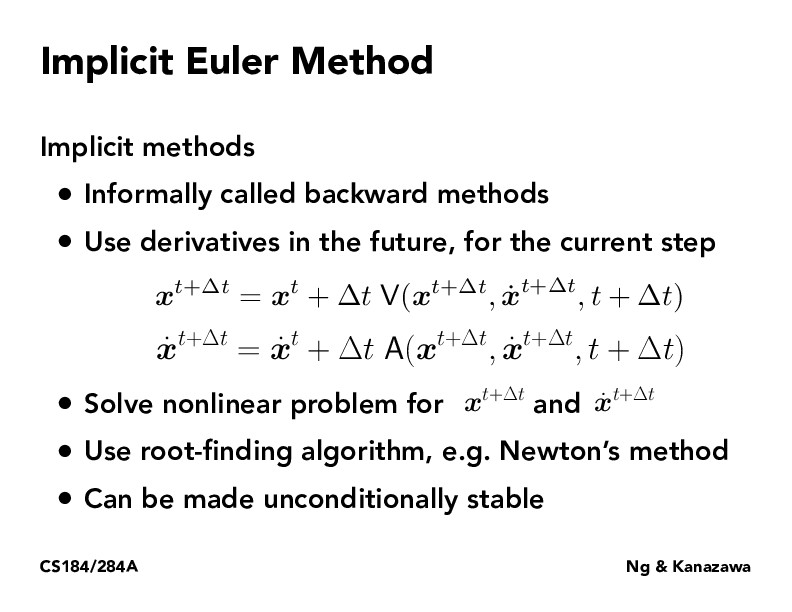Lecture 18: Introduction to Physical Simulation (35)kzhang2

This reminds me of the work here: https://arxiv.org/abs/1909.01377. The authors notice that the output of a transformer is equivalent to the fixed point of a certain operators, which is the solution to an implicit equation. The solution to that implicit equation can be found using a root finding method, like what is presented on this slide. It seems there's a general paradigm of describing dynamical systems using implicit equations and then solving the equations using a root finding method to get the behavior of the system.sutkarsh

@kzhang2 great observation! In general, implicit methods are extremely useful for simulating differential equations, and can work with much larger steps than explicit methods, especially for numerically unstable equations (see https://en.wikipedia.org/wiki/Explicit_and_implicit_methods). These are combined in Runge Kutta methods, which are a popular class of DiffEq solvers

You must be enrolled in the course to comment